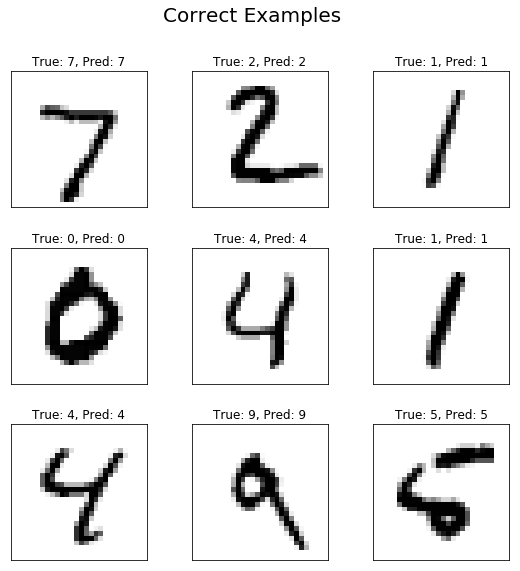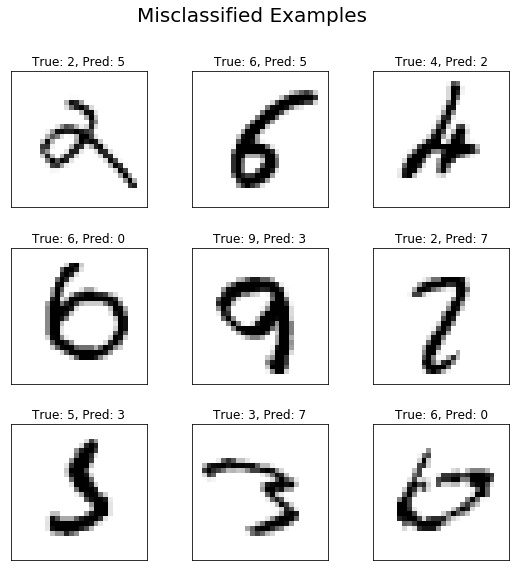# Visualize graph¶

We can visualize our graph using TensorBoard. It helps us to see the conndetion between tensors and how the nodes work.

## Imports:¶

In :
# imports
import tensorflow as tf
import numpy as np
import matplotlib.pyplot as plt


## Input data:¶

For this tutorial we use the MNIST dataset. MNIST is a dataset of handwritten digits. If you are into machine learning, you might have heard of this dataset by now. MNIST is kind of benchmark of datasets for deep learning. One other reason that we use the MNIST is that it is easily accesible through Tensorflow. If you want to know more about the MNIST dataset you can check Yann Lecun's website. We can easily import the dataset and see the size of training, test and validation set:

In :
# Import MNIST data
from tensorflow.examples.tutorials.mnist import input_data

print("Size of:")
print("- Training-set:\t\t{}".format(len(mnist.train.labels)))
print("- Test-set:\t\t{}".format(len(mnist.test.labels)))
print("- Validation-set:\t{}".format(len(mnist.validation.labels)))


Extracting MNIST_data/train-images-idx3-ubyte.gz
Extracting MNIST_data/train-labels-idx1-ubyte.gz
Extracting MNIST_data/t10k-images-idx3-ubyte.gz
Extracting MNIST_data/t10k-labels-idx1-ubyte.gz
Size of:
- Training-set:        55000
- Test-set:        10000
- Validation-set:    5000


## Hyper-parameters:¶

Hyper-parameters are important parameters which are not learned by the network. So, we have to specify them externally. These parameters are constant and they are not learnable.

In :
# hyper-parameters
logs_path = "./logs/visualize_graph"  # path to the folder that we want to save the logs for Tensorboard
learning_rate = 0.001  # The optimization learning rate
epochs = 10  # Total number of training epochs
batch_size = 100  # Training batch size
display_freq = 100  # Frequency of displaying the training results

# Network Parameters
# We know that MNIST images are 28 pixels in each dimension.
img_h = img_w = 28

# Images are stored in one-dimensional arrays of this length.
img_size_flat = img_h * img_w

# Number of classes, one class for each of 10 digits.
n_classes = 10

# number of units in the first hidden layer
h1 = 200


## Graph:¶

Like before, we start by constructing the graph. But, we need to define some functions that we need rapidly in our code. To have an informative graph, we will define a variable_scope for similar operations in our graph. This can be done using tf.variable_scope() function. For example, we can define a variable scope with the name fc1 representing all the nodes in the first fully connected layer.

Notice __with tf.variable_scope().

In :
# weight and bais wrappers
def weight_variable(name, shape):
"""
Create a weight variable with appropriate initialization
:param name: weight name
:param shape: weight shape
:return: initialized weight variable
"""
initer = tf.truncated_normal_initializer(stddev=0.01)
return tf.get_variable('W_' + name,
dtype=tf.float32,
shape=shape,
initializer=initer)

def bias_variable(name, shape):
"""
Create a bias variable with appropriate initialization
:param name: bias variable name
:param shape: bias variable shape
:return: initialized bias variable
"""
initial = tf.constant(0., shape=shape, dtype=tf.float32)
return tf.get_variable('b_' + name,
dtype=tf.float32,
initializer=initial)

def fc_layer(x, num_units, name, use_relu=True):
"""
Create a fully-connected layer
:param x: input from previous layer
:param num_units: number of hidden units in the fully-connected layer
:param name: layer name
:param use_relu: boolean to add ReLU non-linearity (or not)
:return: The output array
"""
with tf.variable_scope(name):
in_dim = x.get_shape()
W = weight_variable(name, shape=[in_dim, num_units])
b = bias_variable(name, [num_units])
layer = tf.matmul(x, W)
layer += b
if use_relu:
layer = tf.nn.relu(layer)
return layer


Now that we have our helper functions we can create our graph.

Same as the scope that we defined for our layers, we can define scopes for our input, loss, optimizer and accuracy.

In :
# Create graph
# Placeholders for inputs (x), outputs(y)
with tf.variable_scope('Input'):
x = tf.placeholder(tf.float32, shape=[None, img_size_flat], name='X')
y = tf.placeholder(tf.float32, shape=[None, n_classes], name='Y')
fc1 = fc_layer(x, h1, 'Hidden_layer', use_relu=True)
output_logits = fc_layer(fc1, n_classes, 'Output_layer', use_relu=False)

# Define the loss function, optimizer, and accuracy
with tf.variable_scope('Train'):
with tf.variable_scope('Loss'):
loss = tf.reduce_mean(tf.nn.softmax_cross_entropy_with_logits(labels=y, logits=output_logits), name='loss')
with tf.variable_scope('Optimizer'):
with tf.variable_scope('Accuracy'):
correct_prediction = tf.equal(tf.argmax(output_logits, 1), tf.argmax(y, 1), name='correct_pred')
accuracy = tf.reduce_mean(tf.cast(correct_prediction, tf.float32), name='accuracy')
# Network predictions
cls_prediction = tf.argmax(output_logits, axis=1, name='predictions')

# Initializing the variables
init = tf.global_variables_initializer()


## Train:¶

As soon as the graph is created, we can run it on a session.

A tf.Session() is as good as it's runtime. As soon as the cell is run, the session will be ended and we will loose all the information. So. we will define an InteractiveSession to keep the parameters for testing.

To write all the summaries on the disk, we use tf.summary.FileWriter class. In this function we will give the path to the folder that we want our logs to be saved. Also, we will pass the graph as sess.graph to visualize our graph in Tensorboard.

In :
# Launch the graph (session)
sess = tf.InteractiveSession() # using InteractiveSession instead of Session to test network in separate cell
sess.run(init)
train_writer = tf.summary.FileWriter(logs_path, sess.graph)
num_tr_iter = int(mnist.train.num_examples / batch_size)
global_step = 0
for epoch in range(epochs):
print('Training epoch: {}'.format(epoch))
for iteration in range(num_tr_iter):
batch_x, batch_y = mnist.train.next_batch(batch_size)
global_step += 1
# Run optimization op (backprop)
feed_dict_batch = {x: batch_x, y: batch_y}
_ = sess.run(optimizer, feed_dict=feed_dict_batch)

if iteration % display_freq == 0:
# Calculate and display the batch loss and accuracy
loss_batch, acc_batch = sess.run([loss, accuracy],
feed_dict=feed_dict_batch)
print("iter {0:3d}:\t Loss={1:.2f},\tTraining Accuracy={2:.01%}".
format(iteration, loss_batch, acc_batch))

# Run validation after every epoch
feed_dict_valid = {x: mnist.validation.images, y: mnist.validation.labels}
loss_valid, acc_valid = sess.run([loss, accuracy], feed_dict=feed_dict_valid)
print('---------------------------------------------------------')
print("Epoch: {0}, validation loss: {1:.2f}, validation accuracy: {2:.01%}".
format(epoch + 1, loss_valid, acc_valid))
print('---------------------------------------------------------')


Training epoch: 0
iter   0:     Loss=2.28,    Training Accuracy=77.0%
iter 100:     Loss=0.40,    Training Accuracy=85.0%
iter 200:     Loss=0.35,    Training Accuracy=93.0%
iter 300:     Loss=0.41,    Training Accuracy=88.0%
iter 400:     Loss=0.27,    Training Accuracy=92.0%
iter 500:     Loss=0.18,    Training Accuracy=95.0%
---------------------------------------------------------
Epoch: 1, validation loss: 0.22, validation accuracy: 93.9%
---------------------------------------------------------
Training epoch: 1
iter   0:     Loss=0.15,    Training Accuracy=97.0%
iter 100:     Loss=0.15,    Training Accuracy=96.0%
iter 200:     Loss=0.11,    Training Accuracy=99.0%
iter 300:     Loss=0.26,    Training Accuracy=95.0%
iter 400:     Loss=0.22,    Training Accuracy=91.0%
iter 500:     Loss=0.16,    Training Accuracy=93.0%
---------------------------------------------------------
Epoch: 2, validation loss: 0.15, validation accuracy: 96.0%
---------------------------------------------------------
Training epoch: 2
iter   0:     Loss=0.11,    Training Accuracy=96.0%
iter 100:     Loss=0.19,    Training Accuracy=93.0%
iter 200:     Loss=0.07,    Training Accuracy=98.0%
iter 300:     Loss=0.15,    Training Accuracy=95.0%
iter 400:     Loss=0.05,    Training Accuracy=99.0%
iter 500:     Loss=0.07,    Training Accuracy=98.0%
---------------------------------------------------------
Epoch: 3, validation loss: 0.12, validation accuracy: 96.7%
---------------------------------------------------------
Training epoch: 3
iter   0:     Loss=0.07,    Training Accuracy=97.0%
iter 100:     Loss=0.08,    Training Accuracy=99.0%
iter 200:     Loss=0.06,    Training Accuracy=98.0%
iter 300:     Loss=0.04,    Training Accuracy=99.0%
iter 400:     Loss=0.08,    Training Accuracy=97.0%
iter 500:     Loss=0.04,    Training Accuracy=100.0%
---------------------------------------------------------
Epoch: 4, validation loss: 0.10, validation accuracy: 97.1%
---------------------------------------------------------
Training epoch: 4
iter   0:     Loss=0.11,    Training Accuracy=97.0%
iter 100:     Loss=0.11,    Training Accuracy=98.0%
iter 200:     Loss=0.13,    Training Accuracy=97.0%
iter 300:     Loss=0.13,    Training Accuracy=93.0%
iter 400:     Loss=0.05,    Training Accuracy=98.0%
iter 500:     Loss=0.05,    Training Accuracy=99.0%
---------------------------------------------------------
Epoch: 5, validation loss: 0.09, validation accuracy: 97.5%
---------------------------------------------------------
Training epoch: 5
iter   0:     Loss=0.06,    Training Accuracy=99.0%
iter 100:     Loss=0.04,    Training Accuracy=99.0%
iter 200:     Loss=0.07,    Training Accuracy=98.0%
iter 300:     Loss=0.04,    Training Accuracy=99.0%
iter 400:     Loss=0.06,    Training Accuracy=99.0%
iter 500:     Loss=0.06,    Training Accuracy=98.0%
---------------------------------------------------------
Epoch: 6, validation loss: 0.08, validation accuracy: 97.6%
---------------------------------------------------------
Training epoch: 6
iter   0:     Loss=0.06,    Training Accuracy=98.0%
iter 100:     Loss=0.06,    Training Accuracy=99.0%
iter 200:     Loss=0.08,    Training Accuracy=98.0%
iter 300:     Loss=0.06,    Training Accuracy=97.0%
iter 400:     Loss=0.02,    Training Accuracy=99.0%
iter 500:     Loss=0.06,    Training Accuracy=98.0%
---------------------------------------------------------
Epoch: 7, validation loss: 0.07, validation accuracy: 97.8%
---------------------------------------------------------
Training epoch: 7
iter   0:     Loss=0.05,    Training Accuracy=98.0%
iter 100:     Loss=0.03,    Training Accuracy=100.0%
iter 200:     Loss=0.05,    Training Accuracy=99.0%
iter 300:     Loss=0.04,    Training Accuracy=99.0%
iter 400:     Loss=0.04,    Training Accuracy=99.0%
iter 500:     Loss=0.04,    Training Accuracy=99.0%
---------------------------------------------------------
Epoch: 8, validation loss: 0.07, validation accuracy: 97.8%
---------------------------------------------------------
Training epoch: 8
iter   0:     Loss=0.01,    Training Accuracy=100.0%
iter 100:     Loss=0.05,    Training Accuracy=98.0%
iter 200:     Loss=0.02,    Training Accuracy=99.0%
iter 300:     Loss=0.08,    Training Accuracy=97.0%
iter 400:     Loss=0.03,    Training Accuracy=99.0%
iter 500:     Loss=0.06,    Training Accuracy=99.0%
---------------------------------------------------------
Epoch: 9, validation loss: 0.08, validation accuracy: 97.6%
---------------------------------------------------------
Training epoch: 9
iter   0:     Loss=0.02,    Training Accuracy=100.0%
iter 100:     Loss=0.01,    Training Accuracy=100.0%
iter 200:     Loss=0.04,    Training Accuracy=98.0%
iter 300:     Loss=0.03,    Training Accuracy=99.0%
iter 400:     Loss=0.02,    Training Accuracy=100.0%
iter 500:     Loss=0.02,    Training Accuracy=99.0%
---------------------------------------------------------
Epoch: 10, validation loss: 0.07, validation accuracy: 97.8%
---------------------------------------------------------


## Test:¶

Now that the model is trained. It is time to test our model.

We will define some helper functions to plot some of the images and their corresponding predicted and true classes. We will also visualize some of the misclassified samples to see why the Neural Net failed to classify them correctly.

In :
def plot_images(images, cls_true, cls_pred=None, title=None):
"""
Create figure with 3x3 sub-plots.
:param images: array of images to be plotted, (9, img_h*img_w)
:param cls_true: corresponding true labels (9,)
:param cls_pred: corresponding true labels (9,)
"""
fig, axes = plt.subplots(3, 3, figsize=(9, 9))
img_h = img_w = np.sqrt(images.shape[-1]).astype(int)
for i, ax in enumerate(axes.flat):
# Plot image.
ax.imshow(images[i].reshape((img_h, img_w)), cmap='binary')

# Show true and predicted classes.
if cls_pred is None:
ax_title = "True: {0}".format(cls_true[i])
else:
ax_title = "True: {0}, Pred: {1}".format(cls_true[i], cls_pred[i])

ax.set_title(ax_title)

# Remove ticks from the plot.
ax.set_xticks([])
ax.set_yticks([])

if title:
plt.suptitle(title, size=20)
plt.show()

def plot_example_errors(images, cls_true, cls_pred, title=None):
"""
Function for plotting examples of images that have been mis-classified
:param images: array of all images, (#imgs, img_h*img_w)
:param cls_true: corresponding true labels, (#imgs,)
:param cls_pred: corresponding predicted labels, (#imgs,)
"""
# Negate the boolean array.
incorrect = np.logical_not(np.equal(cls_pred, cls_true))

# Get the images from the test-set that have been
# incorrectly classified.
incorrect_images = images[incorrect]

# Get the true and predicted classes for those images.
cls_pred = cls_pred[incorrect]
cls_true = cls_true[incorrect]

# Plot the first 9 images.
plot_images(images=incorrect_images[0:9],
cls_true=cls_true[0:9],
cls_pred=cls_pred[0:9],
title=title)

In :
# Test the network after training
# Accuracy
feed_dict_test = {x: mnist.test.images, y: mnist.test.labels}
loss_test, acc_test = sess.run([loss, accuracy], feed_dict=feed_dict_test)
print('---------------------------------------------------------')
print("Test loss: {0:.2f}, test accuracy: {1:.01%}".format(loss_test, acc_test))
print('---------------------------------------------------------')

# Plot some of the correct and misclassified examples
cls_pred = sess.run(cls_prediction, feed_dict=feed_dict_test)
cls_true = np.argmax(mnist.test.labels, axis=1)
plot_images(mnist.test.images, cls_true, cls_pred, title='Correct Examples')
plot_example_errors(mnist.test.images, cls_true, cls_pred, title='Misclassified Examples')


---------------------------------------------------------
Test loss: 0.07, test accuracy: 97.8%
---------------------------------------------------------After we are finished the testing, we will close the session to free the memory.

In :
# close the session after you are done with testing
sess.close()


At this step our coding is done. We have also plotted the accuarcy and some examples. But to inspect more in our network, we can run the Tensorboard. Open your terminal and type:

tensorboard --logdir=logs/visualize_graph --host localhost